How to Subtract in Excel and All Its Formulas & Functions - Compute Expert

# How to Subtract in Excel and All Its Formulas & Functions

Home >> Excel Tutorials from Compute Expert >> Excel Calculations >> How to Subtract in Excel and All Its Formulas & Functions

In this tutorial, you will learn completely how to do subtraction excel calculation. We will also explain a formula you can use to subtract in excel.

The subtraction process in excel can be needed to be done on various data and variables. Numbers, percentages, dates, time, matrixes, and even text are objects that can be subtracted.

We will discuss in detail, how to subtract each object in excel. Besides that, we will also talk about how SUM and the paste special feature can be used as alternative subtraction methods. Hopefully, what you learn here can help you to process data in your excel better!

Disclaimer: This post may contain affiliate links from which we earn commission from qualifying purchases/actions at no additional cost for you. Learn more

## How to Subtract Numbers in Excel

The first material we will discuss in this tutorial is the most basic thing for a subtraction, which is subtracting numbers.

How to do that in excel? Actually, it is pretty easy. We just need to use the help of a dash or minus sign ( - ) in the formula writing on our cells. Input also the number we want to subtract and its subtractor, and we will get the result.

You might know that this is the same way to write a subtraction formula anywhere. It is just, this time, we need to type the formula on our cells in excel.

Generally, here is the writing you need to do in your cell to subtract numbers in excel.

=number_to_subtract - subtractor_number

The numbers you involve in your subtraction calculation can be typed directly or inputted through cell coordinates. Make sure your numbers are in your cells if you want to use cell coordinates in your subtraction formula writing.

To make the explanation much easier to understand, here is the writing practice in excel with its result.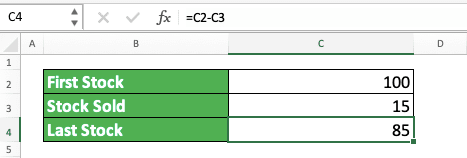As you can see in the example’s formula bar, that is how we usually write an excel subtraction formula. Type the formula correctly in your cell and you will get the result immediately.

This is not too hard, is it? What do you think?

## How to Subtract Percentages in Excel

What if the one you want to become a subtractor is the percentage of the number you want to subtract? There are two methods to do this kind of calculation.

The first one is by getting the subtractor value first before using it to subtract your number. To get the subtractor value, you need to multiply your subtractor percentage with the number you want to subtract.

The general form of the first method’s writing is as follows.

=number_to_subtract - subtractor_percentage * number_to_subtract

Write it in that kind of form and you will get your percentage subtraction result.

Meanwhile, there is also a second method, which is getting the remaining percentage of your number after considering the subtractor percentage. This can be done by subtracting 100 percent (the starting percentage of your number before it is subtracted) with the subtractor percentage. After that, multiply the result with your number.

The general form of the second method’s writing is as follows.

=number_to_subtract*(1 - subtractor_percentage)

One in excel is the same as 100 percent. This is because a percentage number is a number divided by 100 if we write it in a normal number form.

To understand both methods better, here are the examples of their applications in excel.

Method 1:Method 2:As you can see, the two methods give the same result if we write them correctly. So, just choose whichever method that seems easier for you to use.

Note that, if you subtract with a percentage like this, make sure your subtractor percentage is already in a percent form (there is a % symbol behind the number). Or, at least, it is already in its decimal equivalent form so you don’t get the wrong subtraction result (we get the decimal form of a percentage number by dividing its number by 100. For example: 80 percent is the same as 0.8 in decimals (80 divided by 100)).

## How to Subtract Dates in Excel

If you need to subtract dates in your data processing, then the way to do it is pretty easy. You just need to practice the writing to subtract numbers on your dates. However, of course, you must change the numbers with the dates you want to subtract.

To generally illustrate, the writing to subtract dates in excel is as follows.

=later_date - earlier_date

It is the same as writing to subtract numbers in excel, right? Type it in your cell and you will get the day difference of the dates you input in the calculation writing. Don’t forget to put the later date before the earlier date so your formula won’t produce a minus result.

To better understand it, here is an example of dates subtraction in excel with its result.Input your two dates and a minus sign also in one formula writing. As you can see in the example, with that, you will get the date difference that you need.

If you have understood how to subtract numbers in excel, there should be no problem understanding this dates subtraction too!

## How to Subtract Time/Hours in Excel

What if what you want to subtract aren’t dates, but time/hours data? Actually, the way to subtract it is also similar to numbers and dates.

You just need to give a minus sign between your later and earlier time. In writing, here is its general form.

=later_time/hour - earlier_time/hour

Like in the dates subtraction, input the later time first before the earlier time so you won’t get a minus.

The example of the writing usage and its result in excel can be seen below.You can see from the example, the subtraction result is converted automatically by excel into the day unit (the subtraction result in the example is 0.36 days). If you need an hour unit for the result, then you should multiply the result by 24 (because 1 day = 24 hours).

## How to Subtract Days/Months/Years in Excel

If the days/months/years for the difference calculation are in numbers, then you just write as if you subtract numbers. However, if your data are dates and the difference you want isn’t just a day number, then you can use DATEDIF.

DATEDIF, if you haven’t known this formula before, is a formula you can use to look for dates difference. This formula is unique because you won’t see excel suggestions for its writing and inputs when you type it.

With DATEDIF, you can specify the kind of difference you want to get from your dates.

Generally, here is the writing form of DATEDIF.

=DATEDIF(earlier_date, later_date, unit)

In the DATEDIF input, the earlier_date input part becomes the subtractor of the later_date. The unit input there specifies the kind of difference you want to get from your dates.

Here is the list of all the unit inputs you can give in DATEDIF and their brief explanation.

Yyear-
Myearincludes year
Ddayincludes month and year
MDdayexcludes month and year
YMmonthexcludes year
YDdayexcludes year

The meaning of including month and/or year in the additional note column is DATEDIF will calculate those variables if needed. That is, of course, if you use the unit which includes, not excludes, the month and/or year numbers.

As an example, let’s say your earlier date is 12-02-2020 and your later date is 14-05-2021. Your DATEDIF result becomes like this.

If you use an “M” unit input, then the result will be 15. That is because DATEDIF also considers the year numbers from those two dates (the month difference is 3 but because it considers the year difference also which is 1. Because of that, 12 months will be added to the result). But, if you use a “YM” unit input, then the result becomes 3 (because DATEDIF will only calculate the month difference and ignore the year difference).

As an example to make it clearer, here is the writing and result example of DATEDIF in excel.As you can see in the DATEDIF writing example, we use “MD” unit input to calculate the date difference. Because of that, DATEDIF will subtract the day numbers from the two dates only without considering the month and year differences. As a result, the result is 7, which is the difference between 30 and 23 (the day numbers from the two dates).

If you use a “D” or “YD” unit in the DATEDIF, then the result will become much bigger. That is because DATEDIF will also calculate the month and/or year difference too.

If you want to learn deeper about DATEDIF, see the tutorial that discusses the formula specifically here.

## How to Subtract Matrixes in Excel

What if the data we want to subtract in excel is in matrix form? This kind of calculation can be done if you use an array in your subtraction formula.

Generally, here is the writing form of a matrixes subtraction formula in excel.

{=((matrix_cell_range_that_contains_numbers_to_subtract) - (matrix_cell_range_that_contains_subtractor_numbers))}

A matrix that contains numbers is created in Excel using a cell range. To do subtraction which involves two matrixes, we need to input the cell ranges of those two matrixes in our formula. Input the matrix with numbers to subtract first in your formula, before the minus sign.

As you can see in the writing above, there are curly bracket signs at the beginning and end of the formula. Those signs are for the array formula symbol. We need them because we will input cell ranges in a subtraction formula that usually processes individual number inputs.

We use the array formula form by pressing Ctrl + Shift + Enter (Control + Shift + Enter in Mac) buttons after writing the formula. Don’t type the curly bracket signs yourself because that won’t activate the array form in your formula.

If you want to get a matrix result from this, highlight the cell range where you want to put it first. You can see the example in the excel matrixes subtraction process below.After you highlight it, you need to type your matrixes subtraction formula in your active cell in that result cell range. Don’t forget to press Ctrl + Shift + Enter after you finish writing your formula. After that, you will get your matrixes subtraction calculation result!## How to Subtract Columns in Excel

What if the numbers we want to subtract are in one column and we want to get the results fast?

The answer is by writing one subtraction formula in the top row of the column before we copy the formula down. Doing that, you will get the subtraction results of all your column’s numbers much easier.

The writing of the column subtraction formula at the top row is the same as a normal numbers subtraction formula writing.

=number_to_subtract - subtractor_number

To make clearer the things you should do, here is its implementation example in excel. Let’s say we have data like this.We want to get the subtraction results from the first stock and sold stock numbers in each row. How to get them? First, we write a normal numbers subtraction formula in the first row to get its subtraction result.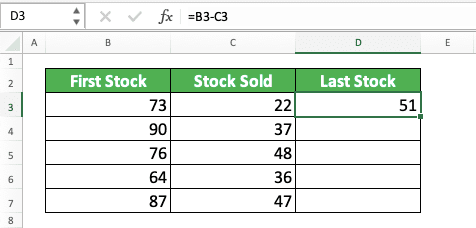After that, move our pointers to the bottom left of our cell cursor on the cell with the subtraction formula. Move it until your pointer becomes a plus sign ( + ) like this.After your pointer changes, click and drag your pointer until the last row of the numbers you want to subtract. You will get your columns’ subtraction result!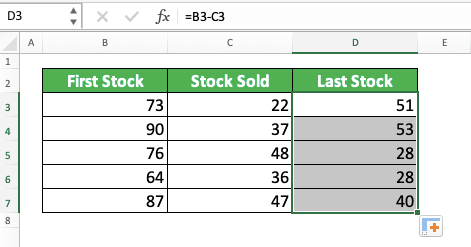Simple, isn’t it? This method is useful if you have a very long column containing the numbers you want to subtract. By copying your formula like that, you can finish your calculation relatively much faster.

## How to Subtract Text in Excel

Sometimes, you also need to subtract text from its particular part so you can get the data processing result you want. Can this be done quickly in excel? It can, of course, by using the combination of TRIM and SUBSTITUTE formulas.

Here is the general writing form of those two formulas combination so you can subtract your text.

=TRIM(SUBSTITUTE(text, part_you_want_to_subtract, “”))

SUBSTITUTE is a formula you use to replace a particular part of a text with something different. However, by writing blank data (“”) in its substitution input part, we can also remove a text part using it.

We also use TRIM here because sometimes there are redundant spaces as a result of removing our text part. With TRIM, the redundant spaces will be deleted automatically.

The example of using the two formulas combination and the implementation’s explanation can be seen below.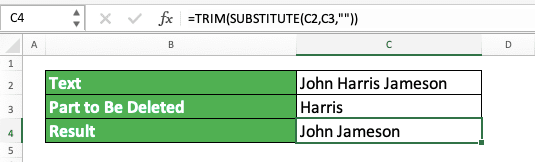In the example, you can see how TRIM and SUBSTITUTE are written to get a text subtraction result. By giving our inputs correctly for those formulas, you can get the text in which the unwanted part has been removed.

If you don’t use TRIM in that example, then there will be two spaces between “John” and “Jameson”. That means there will be one redundant space. Because of that, we use TRIM to remove one of those spaces in the SUBSTITUTE result.

## SUM as a Subtraction Excel Formula

As an alternative method, you can also use SUM as a formula to subtract numbers in excel.

There are two methods to utilize SUM to do a numbers subtraction process in excel. The first one can be used if all your subtractors have been given minus signs in their cells. Besides that, all the numbers you want to calculate are also near to each other in a cell range.

The writing of the first method can be illustrated as follows.

=SUM(numbers_cell_range)

If you have used SUM to sum numbers, then this writing is also similar to that usage. You just need to input the cell range which contains the numbers to subtract and the subtractors in the SUM. Make sure all your subtractor numbers already have minus signs so you won’t get a wrong result.

The second method is if your subtractor numbers are in a particular cell range, separated with the numbers to subtract. The way to write the second method is like this.

=number_to_subtract - SUM(subtractors_cell_range)

If all subtractor numbers in your cell range have minus signs, you need to change the calculation sign with a +.

As an application example of those two methods, you can see the two screenshots below.

Method 1: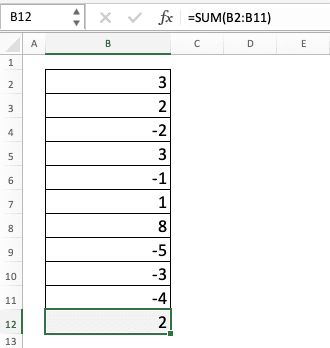Method 2:As suggested by the examples, SUM can subtract your numbers fast if they are in a cell range. If your data fulfills the requirements for a SUM method, then don’t hesitate to use SUM to subtract your numbers easier!

## Paste Special as a Method to Subtract in Excel

There is another thing you can use to subtract numbers in excel, which is the paste special feature. If you want to subtract your numbers fast without writing any formula, maybe this is the best method for you.

Generally, the process to utilize paste special to subtract numbers isn’t hard to do. First, make sure your subtractor number is in a cell that is already in a copy mode. The copy mode is indicated by a dotted line that moves around the cell (you can activate it by pressing Ctrl + C (Command + C in Mac) on your cell).Then, highlight the cell where you place the number you want to subtract. Click the Paste menu dropdown in the Home tab and choose Paste Special…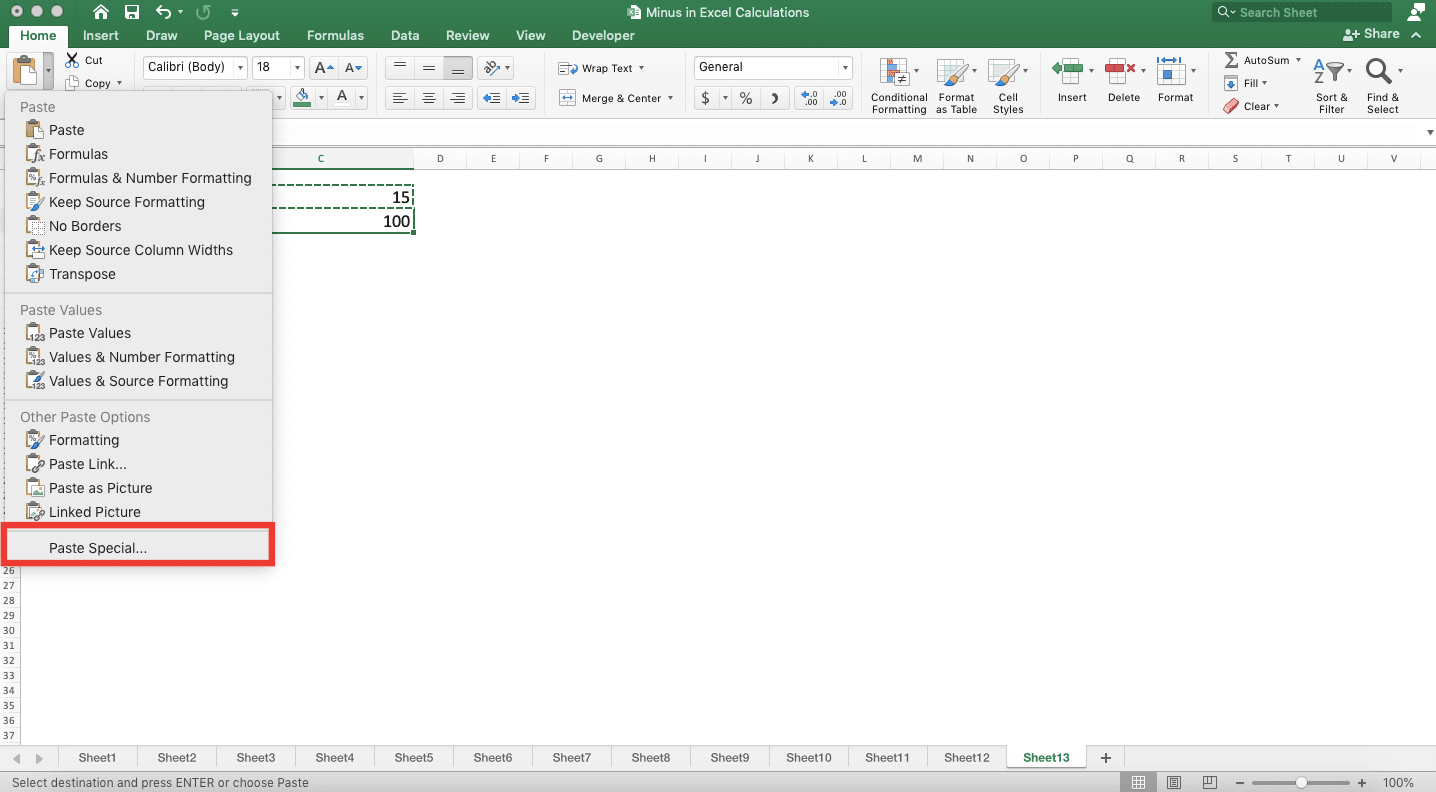In the dialog box shown, in the Operation part, choose the Subtract radio button. Next, click OK.Done! The number you want to subtract has been subtracted by your subtractor, without having to write any formula!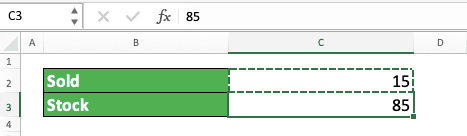## Exercise

After you learn how to subtract various kinds of data in excel, now let’s do an exercise. This is so you can understand better what you have just learned.

### Questions

Answer each question in the appropriate gray-colored cell according to the question number!
1. What is the subtraction result of the number and the percentage?
2. What is the month difference between the two dates? Consider the year numbers too in the process!
3. What is the result if you subtract the text with its subtractor?

Sometimes, you need to make sure your number is positive in your subtraction factor or result. For that, you can use the ABS function in excel. Learn completely about this function through this tutorial!

Related tutorials you should learn:

Get updated excel info from Compute Expert by registering your email. It's free!